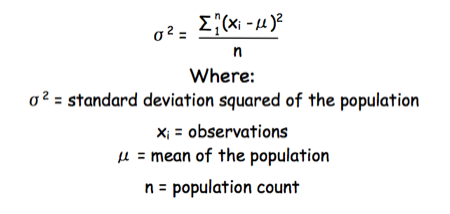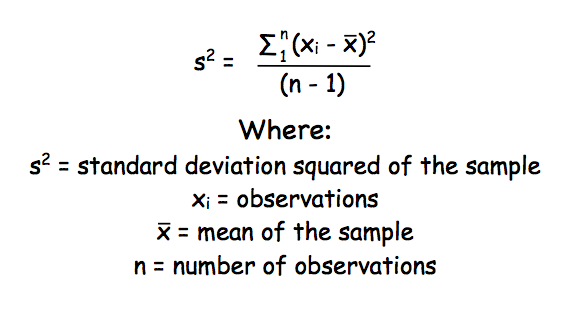## Friday 11 December 2009

### Why did I get my standard deviation calculation wrong?

A number of the class have asked why (or how) they got the standard deviation calculation wrong in the assessment.

Having looked at the class answers it would appear that the main reason students got it wrong is because they were calculating the standard deviation of a POPULATION and not of a SAMPLE.

The equation for the standard deviation of a population (remember a population is ALL possible examples of a measurement/object and is something that is normally difficult to measure - think of the apple example in the lecture - as all examples can't be collected) is:Equation for the standard deviation of a population

Whereas the standard deviation of a sample (which is what we had) is calculated by:Equation for the standard deviation of a sample

Note that the real differences between the two equation are that in the calculation of the standard deviation of a population we are using the true population mean (µ), whereas in the calculation of the standard deviation of a sample we are using an estimate of the mean (x bar), plus in the calculation of the standard deviation of a sample we are using n - 1 (also called degrees of freedom) as this gives better estimate of the true standard deviation of a population (and we are working with a sample).

## Tuesday 1 December 2009

### How many molecules of X are there in a band on a Western Blot?

One of the questions I have often enjoyed setting in western blotting assessments, and that always seems to give problems is....

"You have set up a western blot in which you wish to estimate the amount of GLUT4 per 3T3-L1 adipocyte (the cultured cell line used in the practical). You know that a 35 mm culture dish contains 2 million cells, and gives 1.5 mg total protein. You also have a recombinant stock of GLUT4 at 50 ng/ml. On your gel you load 15 µg of protein from the 3T3-L1 adipocytes and 1, 5, 10 and 20 µl of the GLUT4 stock. After probing with antibodies you have a 55 kDa band in the 3T3-L1 adipocyte lane, and in the GLUT4 stock lane. The band in the 3T3-L1 adipocyte sample has the same intensity of band as the 10 µl GLUT4 stock. How many molecules of GLUT4 are there per adipocyte?"

The chances are you are getting 'freaked out' by all the weird numbers flying around. The answer is to go back to basics.

For example:

If you had something with a molecular weight of 10, and you loaded 5 g on a lane, you would have 0.5 moles in that lane.

You know how many molecules there are in a mole (it is a constant that is the number of atoms in 12 g of carbon 12), so that constant multiplied by the number of moles, would be the number of molecules in the lane.

Let's say 1 mole of something contains 100 molecules (which it doesn't, the real number is much much bigger).

So, if you have 0.5 mole in the lane you would have 50 molecules in that lane.

If you have another lane with a known number of cells in, say 25, and that lane has the same intensity of staining as the lane containing 50 molecules you could say that lane also has 50 molecules.
These 50 molecules came from 25 cells, so each cell must have 2 molecules.....

Now you can work it through with 'simple' numbers all you need to do is to plug in the 'complicated' numbers in the question....

If you struggle with 'Science Maths' then you may like to look at: Maths4Biosciences